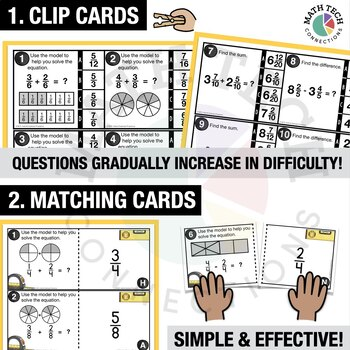# 4th - Add & Subtract Fractions Math Centers - Math GamesSubject
Resource Type
File Type
Zip (12 MB|30 pages)
Standards
\$4.00
Also included in:
1. These Fourth Grade math games will save you time and help students stay focused and organized during math centers. This resource includes 24 game sets. This bundle includes a total of 72 math games, so you will have math centers for the entire year!CLICK HERE TO DOWNLOAD ONE FREE SET!★ Each game set
\$92.00
\$50.00
Save \$42.00
• Product Description
• Standards

These Add & Subtract Fractions math games will save you time and help students stay focused and organized during math centers.

Learning Goals: I can understand addition and subtraction of fractions as joining and separating parts referring to the same whole. I can decompose a fraction into a sum of fractions with the same denominator. I can add and subtract mixed numbers with the same denominator.

What's Included?

- 3 Math Games: Math Sort, Matching Game, Clip Cards

- Recording Sheet

- Getting Started Directions

- Label Cards for Organization

The Problem with Guided Math

- One big problem with guided math is not knowing what to assign for math centers.

- It can get especially overwhelming when pulling math games from various places. Teachers end up wasting lots of time explaining directions and students get off task because they are confused.

- Another big problem is holding students accountable for their work during math centers. You want students to record their work, but then you don’t have extra time to check or organize all the recording sheets.

The Solution

✓ The math games are standards-aligned. This means you know students are practicing the skills you are required to teach. The learning goal is posted on each math game.

✓ There is a predictable routine. Each math center has 3 math games per math standard. (Math Sort, Matching Game, Clip Cards) The game structure is the same, so you won’t waste time teaching new games each week.

✓ Students use one recording sheet. Students stay organized by only needing one recording sheet per topic. An answer key is provided, so you or students can check their answers.

✓ These math games come with label cards that will help you organize the math centers. These label cards include pictures and directions for each game.

Math Standard

CCSS: 4.NF.3: I can understand addition and subtraction of fractions as joining and separating parts referring to the same whole. I can decompose a fraction into a sum of fractions with the same denominator. I can add and subtract mixed numbers with the same denominator.

Correlates to //

TEKS:

MAFS:

More MATH GAMES in this Series

Solve word problems involving addition and subtraction of fractions referring to the same whole and having like denominators, e.g., by using visual fraction models and equations to represent the problem.
Add and subtract mixed numbers with like denominators, e.g., by replacing each mixed number with an equivalent fraction, and/or by using properties of operations and the relationship between addition and subtraction.
Decompose a fraction into a sum of fractions with the same denominator in more than one way, recording each decomposition by an equation. Justify decompositions, e.g., by using a visual fraction model. Examples: 3/8 = 1/8 + 1/8 + 1/8; 3/8 = 1/8 + 2/8; 2 1/8 = 1 + 1 + 1/8 = 8/8 + 8/8 + 1/8.
Understand addition and subtraction of fractions as joining and separating parts referring to the same whole.
Understand a fraction 𝘢/𝘣 with 𝘢 > 1 as a sum of fractions 1/𝘣.
Total Pages
30 pages